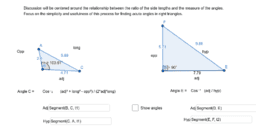# APS Geometry Unit 4 Book

Topic:
Geometry
MGSE9-12.G.SRT.6 Understand that by similarity, side ratios in right triangles are properties of the angles in the triangle, leading to definitions of trigonometric ratios for acute angles. MGSE9-12.G.SRT.7 Explain and use the relationship between the sine and cosine of complementary angles. MGSE9-12.G.SRT.8 Use trigonometric ratios and the Pythagorean Theorem to solve right triangles in applied problems.